# Logarithmic Functions

Parent topic:
Functions
•### Logarithmic Functions: Graphs

Activity

Tim Brzezinski

•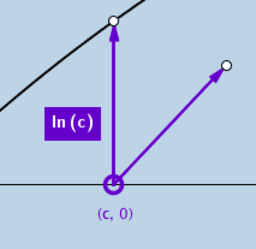### Logarithmic Action (3)!

Activity

Tim Brzezinski

•### Logarithmic Action (4)!

Activity

Tim Brzezinski

•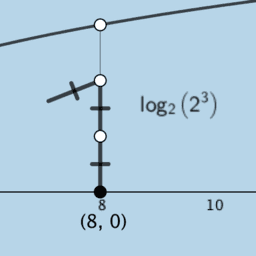### Logarithmic Action (4)! V2

Activity

Tim Brzezinski

•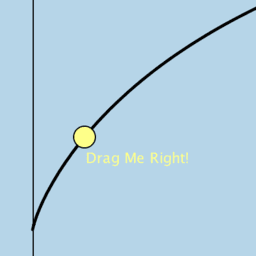### One Special Limit (V2)

Activity

Tim Brzezinski

•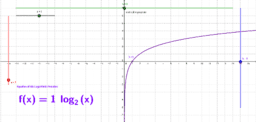### Logarithmic Functions (Graph & Equation Anatomy)

Activity

Tim Brzezinski

•### Exponential & Logarithmic Functions

Book

Tim Brzezinski

•### Logarithmic Action (3: V2)

Activity

Tim Brzezinski

•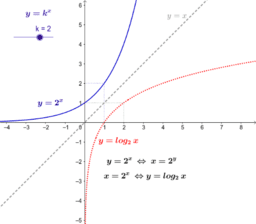### Exponential & logarithmic functions

Activity

Gerrit Stols

•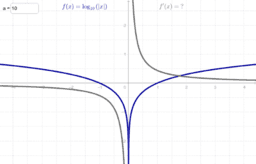### Derivative of logarithmic function

Activity

•### The touches of y=a^x and y = log{a} x

Activity

Anthony OR 柯志明

•### Exponential & Logarithmic Functions

Book

SAVAŞ ORHAN

•### Exponential and logarithmic functions

Activity

Juan Carlos Ponce Campuzano

•### Exponential and Logarithmic Functions

Book

Whit Ford

•### Graphs of Logarithmic Functions 對數函數的圖像

Activity

Alex CHIK

•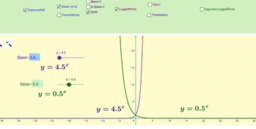### Exponential and logarithmic function.

Activity

Luis Miguel López Herranz

•### Practice with steps: Solving Log Eqns (Product/Quotient law)

Activity

Hisham Amir

•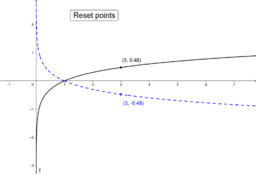### log (x) vs. log (1/x)

Activity

mcdull

•### Transformation of Logarithmic Functions

Activity

Math Destroyer

•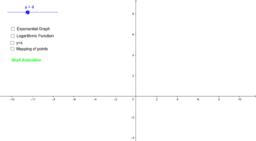### Exponential and Logarithmic Functions

Activity

Manjeet Kaur

•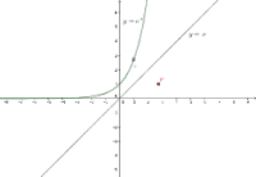### Reflecting the Exponential Function

Activity

Guillermo Bautista

•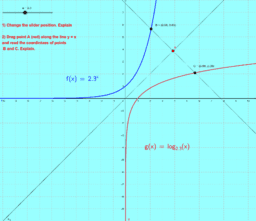### Exponential and Logarithmic Functions

Activity

•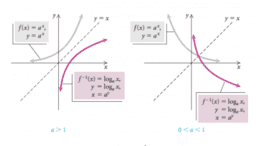### Exploring Exponential & Logarithmic Functions

Book

Jeremias Endrina Jr

•### Exploring Exponential & Logarithmic Functions

Book

Mina Sedaghatjou